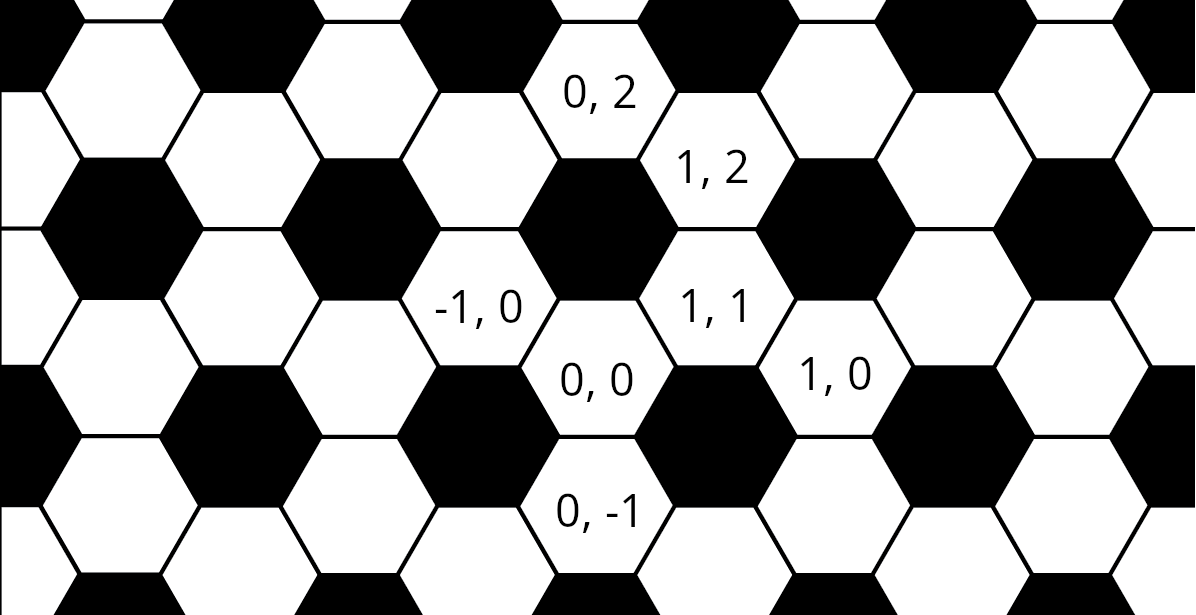# Jane Street Puzzle, July 2022: Andy’s Morning Stroll

In this problem, we shall compare the return times of random walks on two different graphs.Andy the ant has spent most of his days living on a strange land consisting of white hexagons that are surrounded by alternating black pentagons and white hexagons (three of each), and black pentagons surrounded by five white hexagons. To us this land is familiar as the classic soccer ball we see above on the left. Due to Andy’s tiny size and terrible eyesight, he doesn’t notice the curvature of the land and avoids the black pentagons because he suspects they may be bottomless pits.
Every morning he wakes up on a white hexagon, leaves some pheromones to mark it as his special home space, and starts his random morning stroll. Every step on this stroll takes him to one of the three neighboring white hexagons with equal probability. He ends his stroll as soon as he first returns to his home space. As an example, on exactly 1/3 of mornings Andy’s stroll is 2 steps long, as he randomly visits one of the three neighbors, and then has a 1/3 probability of returning immediately to the home hexagon.
This morning, his soccer ball bounced through a kitchen with an infinite (at least practically speaking…) regular hexagonal floor tiling consisting of black and white hexagons, a small part of which is shown above on the right. In this tiling every white hexagon is surrounded by alternating black and white hexagons, and black hexagons are surrounded by six white hexagons. Andy fell off the ball and woke up on a white hexagon. He didn’t notice any change in his surroundings, and goes about his normal morning routine.
Let p be the probability that his morning stroll on this new land is strictly more steps than the expected number of steps his strolls on the soccer ball took. Find p, rounded to seven significant digits.

# Random walk on the soccer ball (truncated icosahedron)

We firstly compute the mean return time of a random walk on the soccer ball.

## Stationary distribution

Let $0, ..., 19$ be the vertices of the underlying soccer ball graph and $\pi_i$ be its stationary distribution ($\pi_i$ is the asymptotic probability to be in vertex $i$ in a random walk).

The soccer ball being totally symmetric (it is a vertex-transitive graph), its stationary distribution is uniform: $$\pi := \begin{pmatrix} \pi_0\\ \vdots\\ \pi_{19} \end{pmatrix} = \frac{1}{20}\begin{pmatrix} 1\\ \vdots\\ 1 \end{pmatrix}$$

## Mean return time

Let $P = (p_{i, j})_{0\leq i\leq 19, ~0\leq j\leq 19}$ be the transition matrix of the soccer ball graph. Here, $p_{i, j} = 1/3$ if $i$ and $j$ are adjacent vertices ($p_{i, j} = 0$ otherwise).
Let $E = (E[T_{i, j}])_{0\leq i\leq 19, ~0\leq j\leq 19}$ be the hitting time matrix: $E[T_{i, j}]$ is the expected number of steps to reach vertex $j$ from vertex $i$.

Theorem: $$E[T_{i, i}] = \frac{1}{\pi_i}$$

Proof:
A path from $i$ to $j$ can be split into a step to a neighbor $k$ of $i$ and a path from $k$ to $j$. Therefore: $$E[T_{i, j}] = 1 + \displaystyle\sum_{k \neq j} p_{i, k} E[T_{k, j}] \quad (*)$$ In a matrix form: $$E = U + PE - P\Delta$$ where $U$ is filled with $1$ and $\Delta = \text{diag}(E[T_{0, 0}], ..., E[T_{19, 19}])$.

Let's multiply by $\pi$ on the left: $$\pi^T E = \pi^T U + \pi^T PE - \pi^T P\Delta$$

Since $\pi$ is stationary, $\pi^T P = \pi^T$.
Therefore: $$\pi^T \Delta = \pi^T U = \begin{pmatrix} 1 \\ \vdots \\ 1 \end{pmatrix}$$ $$\pi_i E[T_{i, i}] = 1$$ $$\boxed{E[T_{i, i}] = \frac{1}{\pi_i}}$$

Remark: This theorem holds for any irreducible (strongly connected) Markov chain.

Applied to our problem, this theorem shows that Andy's mean return time is $20$ on the soccer ball.

# Random walk on the kitchen

It looks more difficult to mathematically find the mean return time in the second case. Instead, we will compute it using dynamic programming on induction formula similar to $(*)$.
We use the following coordinates to identify each vertex:In :
from functools import cache

@cache
def proba(i, j, n):
""" Probability of not reaching (0, 0) from (i, j) in 20 - n steps """
if n > 20:
return 1
if i == 0 == j and n > 0:
return 0

p1 = proba(i - 1, j, n + 1) # left cell
p2 = proba(i + 1, j, n + 1) # right cell
p3 = proba(i, j + (1 if n % 2 == 1 else -1), n + 1) # up or bottom cell
return (p1 + p2 + p3)/3

float(f"{proba(0, 0, 0):.7f}")

Out:
0.4480326

Tags:

Updated: